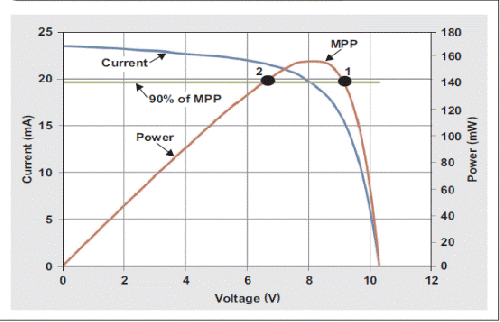Feb. 22, 2019
Share:

# What you need to know When it comes to Choose solar panel and solar inverter for pump

## Open Circuit Voltage (Voc)

Open circuit voltage is how many volts the solar panel outputs with no load on it.

This is a very important number, as it is the maximum voltage that the solar panel can produce under standard test conditions, so this is the number to use when determining how many solar panels you can wire in series going into your inverter or charge controller.

Maximum Power Point (Pmax)

The Pmax is the sweet spot of the solar panel power output, located at the “knee” of the curves in the graph above. It is where the combination of the volts and amps results in the highest wattage (Volts x Amps = Watts)Maximum Power Point Current (Impp)

he Impp is the current (amps) when the power output is the greatest. It is the actual amperage you want to see when it is connected to the MPPT solar equipment under standard test conditions.

Maximum Power Point Voltage (Vmpp)

The Vmpp is the voltage when the power output is the greatest. It is the actual voltage you want to see when it is connected to the MPPT solar equipment (like an MPPT solar inverter) under standard test conditions.

When you use a Maximum Power Point Tracking MPPT) charge controller or inverter, this is the point that the MPPT electronics tries to keep the volts and amps at to maximize the power output. The wattage that a solar panel is listed as is the Pmax where Pmax = Vmpp x Impp

Second,Select correct modes of solar pump inverter (Take KEWO SG320 Series Solar Pump Inverter as exemple)

Voltage, power and current) as pumps nameplate and field requirement.

Get field requirement more in detail, such as water head, water flow, distance from pump to inverter, pumps voltage, pumps rated current, maximum current of pumps, and working conditions.

The selecting rated current of inveter must be equal or bigger than rated of using pump.

The bigger power of inverter should be selected for long distance from pumps to inverter. As experience, at least request 1.3 times bigger power input of solar arrays compare to rated power of pumps. It is also much related with the quality and efficiency of solar panels.

Input voltage, total power solar arrays selection

 Input voltage, power solar arrays selection Pumps model Inverte models Vmp Voc Total Power of solar arrays 110VAC pumps 1S 110*1.41=130VDC 156VDC ≧ (1.3 to 2.0) rated power of pumpsIt is also depend on the quality of solar panels. The more power input, the better performance. 220VAC pumps 2S 220*1.41=310VDC 372VDC 380VAC pumps 4T (Max 800VDC) 380*1.41=540VDC 648VDC 480VAC pumps 4T ( Max 900VDC) 480*1.41=677VDC 812VDC

Although SG320 inverter built in high efficiency MPPT tracking arithmetic, up to 99.2% MPPT efficiency, but also need to consider many factors of solar radiation. It is no certain rules to determine exactly how many piece solar panels exactly input for system. If the output frequency can’t get to 50Hz/60Hz, consider to connect more solar panels.

Solar arrays selection table for inverter and pumps.

Take solar panels Voc38VDC, Vmp 31VDC, 265W. Check if enough DC voltage for system, Voc and Vmp. If the input is incorrect, the inverter will not work properly. If the output frequency is not high, lower than 50/60Hz even in good sunlight radiation, check if enough solar panels have been connected. The total power should be at least bigger 1.3 times than of rated power of pumps.

 265w, 38Voc (Open circuit voltage), 31Vmp ( Voltage at Pmax) Inverter models Power of pump Connection in series (PCS) (Vmp) Connect in parallel ( Strings) Power Total (PCS) 1S (110VAC) 0.75kw to 1.0kw 4 or 5 PCS 1* strings 5*1=5 2S (220VAC) 0.75kw to 1.5kw 10PCS 1* strings 10*1=10 2S (220VAC), Max 450vdc 2.2kw 11PCS 1* strings 11*1=11 4T(380VAC) 0.75kw to 2.2kw 18PCS 1* strings 18*1=18 4T(380VAC)Max 900VDC 3.7kw 20PCS 1* strings 20*1=20 4T(380VAC) 5.5kw 18PCS 2* strings 18*2=36 4T(380VAC)Max 900VDC 7.5kw 20pcs 2* strings 20*2=40 4T(380VAC) 11kw 18pcs 3* strings 18*3=54 4T(380VAC)Max900VDC 15kw 20pcs 4* strings 20*4=80